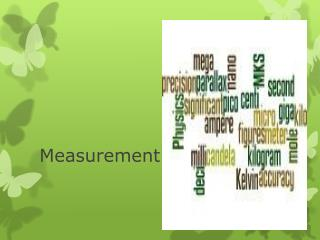DownloadDownload PresentationMeasurement

# Measurement

Download Presentation## Measurement

- - - - - - - - - - - - - - - - - - - - - - - - - - - E N D - - - - - - - - - - - - - - - - - - - - - - - - - - -
##### Presentation Transcript

1. Measurement

2. Physics • A branch of science that involves the study of the physical world: energy, matter, and how they are related

3. Physics • In physics, equations are important tools for modeling observations and for making predictions. • Mathematics is the language of physics.

4. Measurements The Système International d’Unités, or SI, uses seven base quantities, which are shown in the table below.

5. SI • The base quantities were originally defined in terms of direct measurements. Other units, called derived units, are created by combining the base units in various ways. Ex. Speed (m/s), Newton (kg m/s2), Volume (cm3) • The SI system is regulated by the International Bureau of Weights and Measures in Sèvres, France. • This bureau and the National Institute of Science and Technology (NIST) in Gaithersburg, Maryland, keep the standards of length, time, and mass against which our metersticks, clocks, and balances are calibrated.

6. SI • Prefixes are used to change SI units by powers of 10, as shown in the table below.

7. MKS • The MKS system of measurement is used in physics. • MKS stands for meters, kilograms, seconds • All measurements must be converted to meters, kilograms, and seconds

8. Measurements • A measurement is a comparison between an unknown quantity and a standard. • Measurements quantify observations. • Careful measurements enable you to derive the relation between any two quantities.

9. Precision and Accuracy • Precision depends upon the instrument being used. • How small the units are in the measuring device • How close several measurements are to each other. • Accuracy is how close the measurement is to the known or actual value. anim1-2_index.html

10. Another definition of Precision

11. Techniques of Good Measurement • Scales should be read with one’s eye directly above the measure. • If the scale is read from an angle, as shown in figure (b), you will get a different, and less accurate, value. • The difference in the readings is caused by parallax, which is the apparent shift in the position of an object when it is viewed from different angles • Always read a measurement to the precision of the instrument mov/ch1_scn36_smallanim.mov

12. Scientific Notation • Scientific notation is used in science to make it easier to use very large or very small numbers easily or for showing significant figures • Numbers in scientific notation are always written with one number to the left (may have unlimited behind the decimal) of the decimal point and a power of 10. • Makes converting easier • Positive exponents mean the number is larger than 1 and negative exponents means the number is smaller than 1 • 3.65 x 103= 3650 and 3.65 x 10-3 = 0.00365

13. Significant Figures • In making measurements, the last digit is always estimated in between the smallest division of the scale. • All digits known for certain and the last estimated digit in a measurement are significant.

14. Significant Figures • Significant figures are important for us to know how to round off our answers when we calculate. • A calculated answer can never be any more precise than the least precise measurement. • When we calculate, especially multiply and divide, the decimal places are often increased. • We must round our answer to an appropriate point. • The use of significant figures helps us do that.

15. Significant Digits • All non-zero digits are significant. Ex. 384.3, 56.7, 9993.223 • Final zeros behind the decimal are significant because they show precision of the instrument. Ex. 4.00 cm, 6.7890 g, 3.4000 mm • Zeros used only for placing the decimal are not significant. Ex. 4000 km, 20 g, 0.00348 mm, 0.0654 L • Zeros between two other significant digits are significant. Ex. 405 g, 6007 moles, 78.099 L

16. Calculations involving rounding • The use of significant digits can help tell us where to round our answers. • When multiplying or dividing, round to the least number of significant figures in the problem. Ex. #1 (3.21 cm x 2.2 cm = 7.062 cm3) Ex. #2 (54.6 cm x 3.12 cm x 2.7 cm = 459.9504 cm3 ) so we round to 460 cm3 • When adding and subtracting, round the least number of decimal places. Ex. 89.0 mm + 45.609 mm + 34.90 mm = 169.509 mm so round to least precise measurement = 169.5 mm